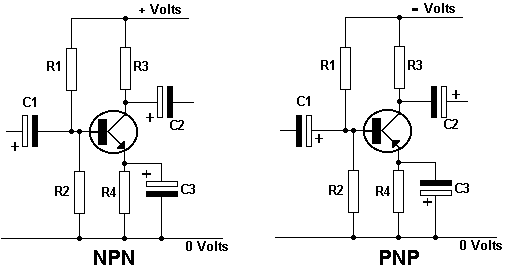Home > Electronic Tutorials > Junction Transistors Tutorial > Biasing a Transistor Tutorial

Junction Transistors Electronic Tutorials

# Biasing a Transistor TutorialChoose a general purpose transistor with a beta gain higher than 100. Decide on the collector current.

The base bias voltage is be 1/3 of the supply voltage.  The current through the base bias potential divider is to be 1/10 of the collector current.

Calculate the two base resistor values, R1 and R2. The emitter voltage is 0.6 volts lower than the base voltage. The value of the emitter resistor R4 is the emitter voltage divided by the collector current. The value of the collector resistor R3 is the supply voltage divided by three times the collector current. The values of the capacitors depend upon the application. Study a few circuits.

For common collector and common base some of the capacitors are connected differently.